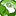# New to Qlik Sense

If you’re new to Qlik Sense, start with this Discussion Board and get up-to-speed quickly.

cancel
Showing results for
Did you mean:Contributor III

## Set analysis to determine percentage which adjusts for time dimension

Not really sure how to explain this but here goes.

I have a calculation which sums up the number of non-automated jobs:

``Sum({<[Failure Code-reason]-={'Success'}>}[jobs])``

I can calculate the percentage of non-automated jobs with the following:

``````Sum({<[Failure Code-reason]-={'Success'}>}[jobs])
/
(Sum({1<[Failure Code-reason]-={'Success'}>}[jobs]))``````

I want to be able to calculate these for a where a user is interacting with a lot of filters except where a user selects a time dimension called [Mon].

The purpose of this is to always calculate percent of failures over the selected time period.

I can't figure out a way to evaluate the above based on the [Mon] selection

Any ideas?

Labels (1)
• ### Set Analysis

1 Solution

Accepted SolutionsContributor III
Author

By simplifying the problem I was able to figure this one out through searching the forums.

The following formula works for my purpose:

``````(Sum({<[Mon]=P([Mon])>*<[Failure Code-reason]-={'Success'}>}[jobs]))
/
(sum({1<[Mon]=P([Mon])>*1<[Failure Code-reason]-={'Success'}>} TOTAL [jobs]))``````

Cheers,

Thomas

4 RepliesCreator

Call that into the expression, as follows

``````Sum({<[Failure Code-reason]-={'Success'}, Time-={'Mon'}>}[jobs])
/
(Sum({1<[Failure Code-reason]-={'Success'}, Time-={'Mon'}>}[jobs]))``````Contributor III
Author

Thanks for your response Saminea, but it isn't working for me.

Expected result is where a filter on only [Mon], the value should stay as 1 (100%) because it reflects 100% of the jobs for the [Mon]

When filtered on any other dimension e.g. [Client], [Stream] etc. it should then break down to a value less than 1 based on the % of failure jobs for teh selected [Mon] (or multiple).

Where I select one more multiple [Mon], your formula like mine is still evaluating the percentage of total i.e. 0.07 (7%)

Any ideas?Contributor III
Author

Still stuck on this one. I think the issue here is the denominator.

`(Sum({1<[Failure Code-reason]-={'Success'}, Time-={'Mon'}>}[jobs]))`

The formula will work if I can get this to:

1. Where [Mon] (Master Calendar Dimension) is selected, update the number based on the filter;
2. When other dimensions are selected, disregard selections

I suspect this isn't too hard and I'm just missing something!Contributor III
Author

By simplifying the problem I was able to figure this one out through searching the forums.

The following formula works for my purpose:

``````(Sum({<[Mon]=P([Mon])>*<[Failure Code-reason]-={'Success'}>}[jobs]))
/
(sum({1<[Mon]=P([Mon])>*1<[Failure Code-reason]-={'Success'}>} TOTAL [jobs]))``````

Cheers,

ThomasTags
Community Browser